Examples

Chapter 3 Class 11 Trigonometric Functions
Serial order wise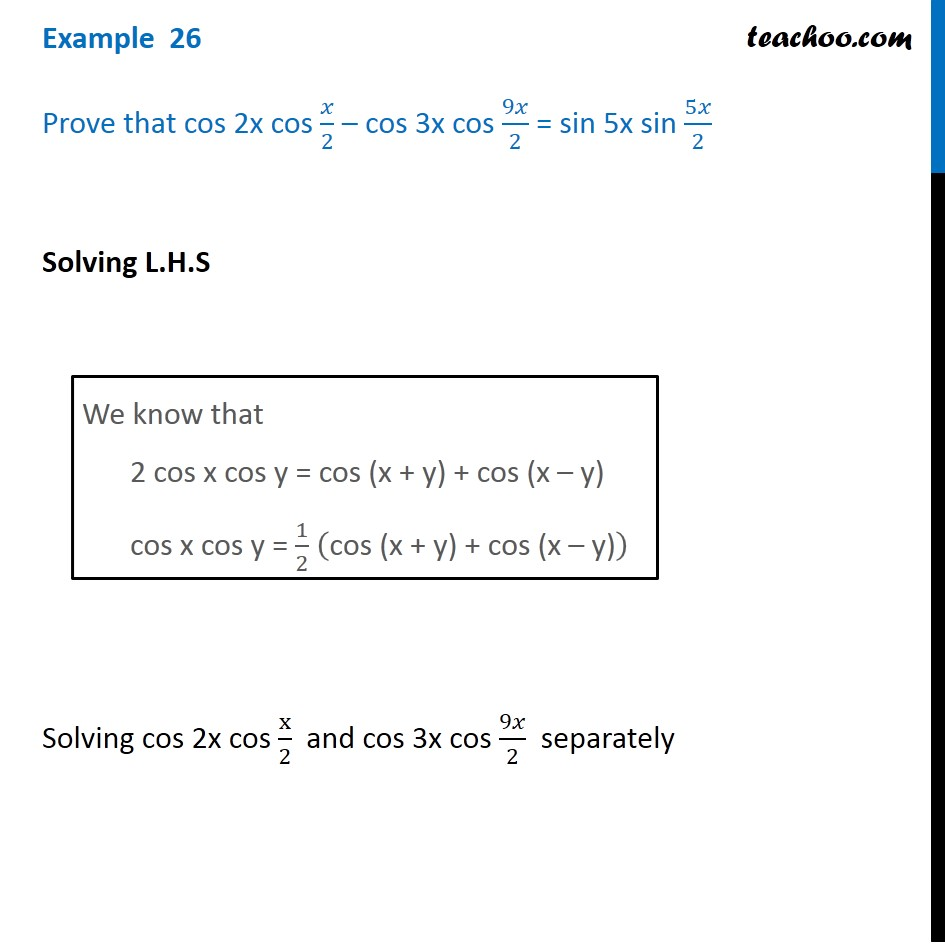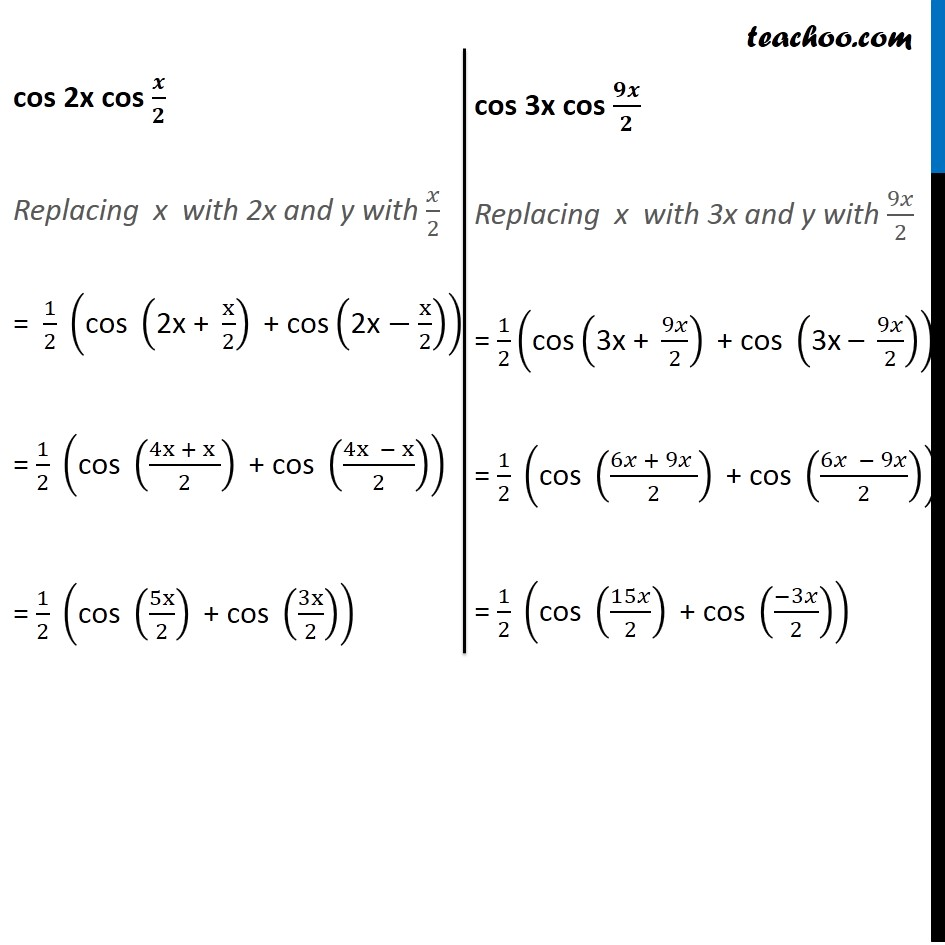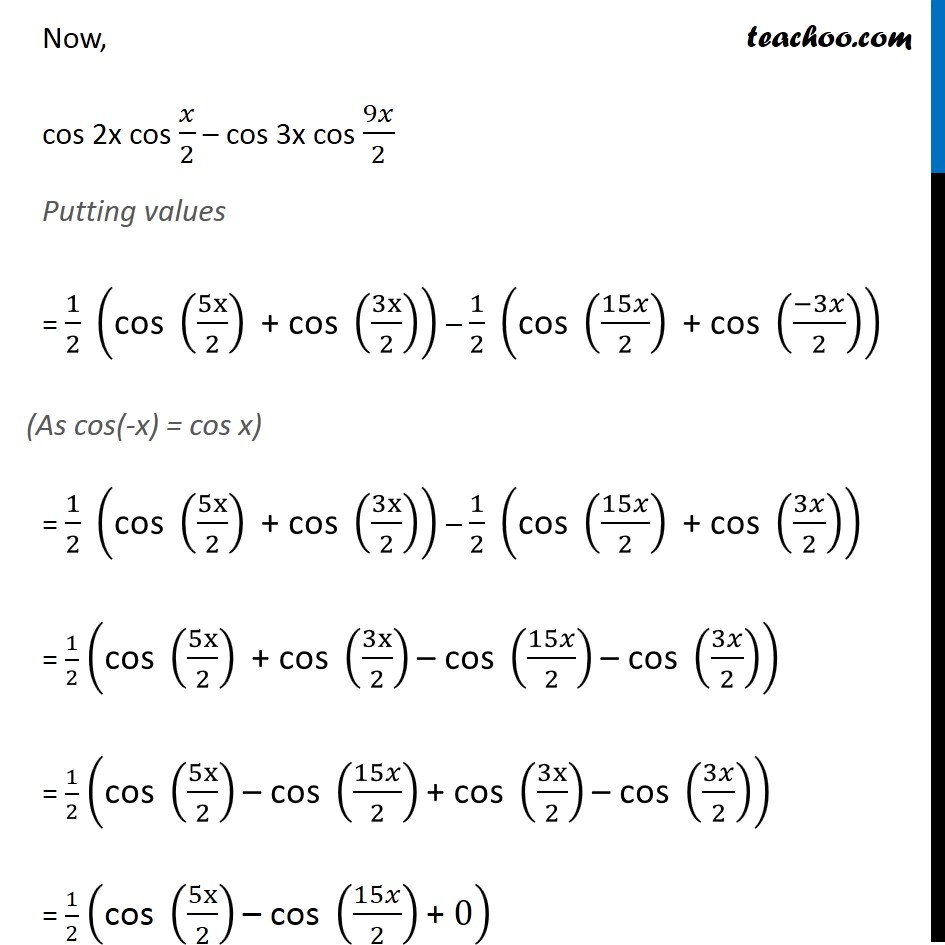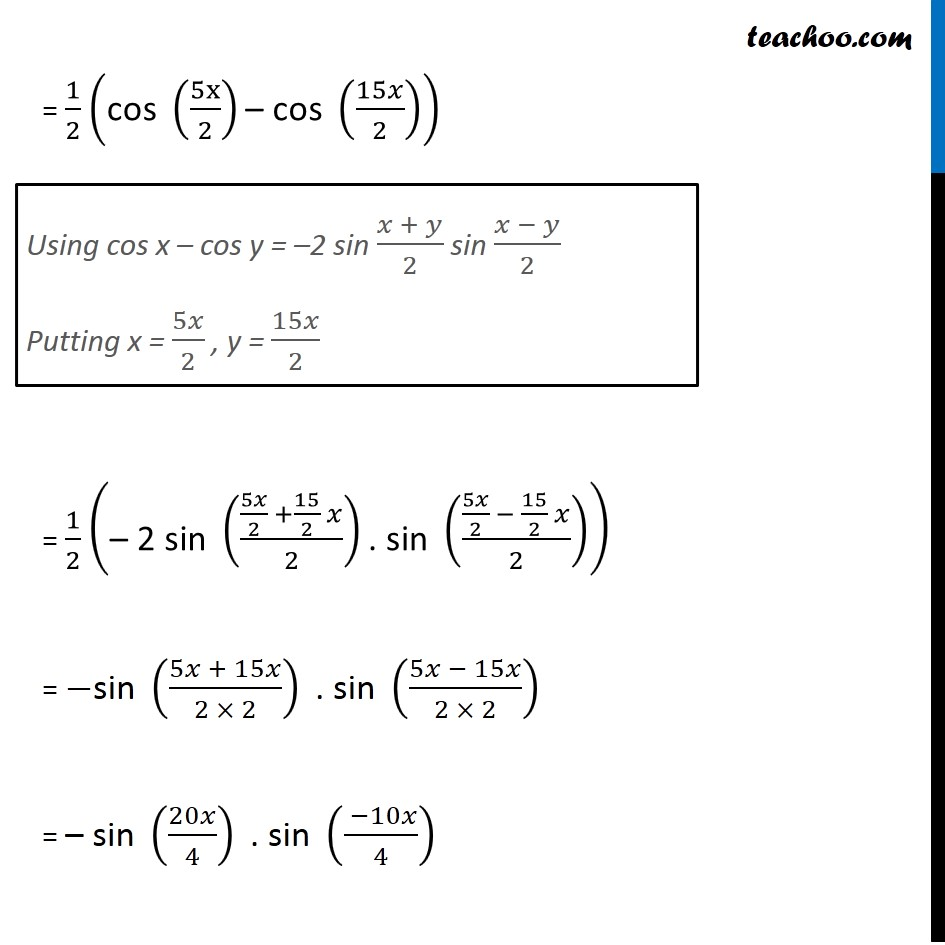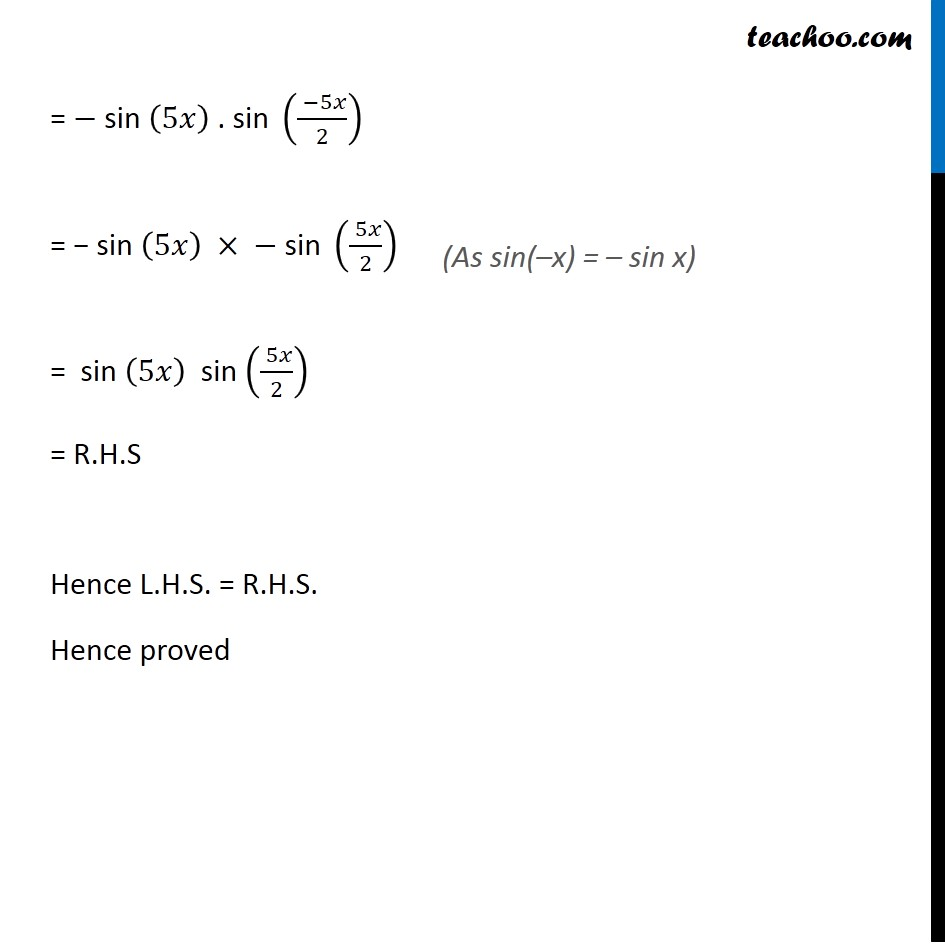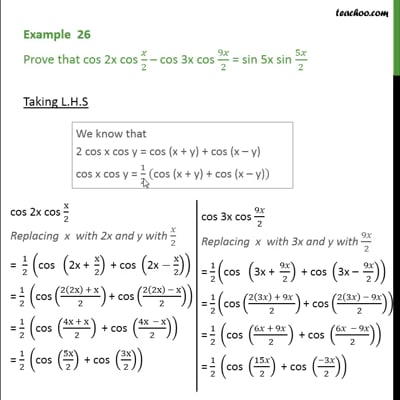This video is only available for Teachoo black users

Maths Crash Course - Live lectures + all videos + Real time Doubt solving!

### Transcript

Example 26 Prove that cos 2x cos 𝑥/2 – cos 3x cos 9𝑥/2 = sin 5x sin 5𝑥/2 Solving L.H.S Solving cos 2x cos x/2 and cos 3x cos 9𝑥/2 separately We know that 2 cos x cos y = cos (x + y) + cos (x – y) cos x cos y = 1/2 ("cos (x + y) + cos (x – y)" ) cos 2x cos 𝒙/𝟐 Replacing x with 2x and y with 𝑥/2 = 1/2 ("cos " ("2x + " x/2)" + cos" ("2x" −x/2)) = 1/2 ("cos " ((4x + x )/2)" + cos " ((4x − x)/2)) = 1/2 ("cos " (5x/2)" + cos " (3x/2)) cos 3x cos 𝟗𝒙/𝟐 Replacing x with 3x and y with 9𝑥/2 = 1/2 ("cos" ("3x + " 9𝑥/2)" + cos " ("3x – " 9𝑥/2)) = 1/2 ("cos " ((6𝑥 + 9𝑥 )/2)" + cos " ((6𝑥 − 9𝑥)/2)) = 1/2 ("cos " (15𝑥/2)" + cos " ((−3𝑥)/2)) Now, cos 2x cos 𝑥/2 – cos 3x cos 9𝑥/2 Putting values = 1/2 ("cos " (5x/2)" + cos " (3x/2)) – 1/2 ("cos " (15𝑥/2)" + cos " ((−3𝑥)/2)) = 1/2 ("cos " (5x/2)" + cos " (3x/2)) – 1/2 ("cos " (15𝑥/2)" + cos " (3𝑥/2)) = 1/2 ("cos " (5x/2)" + cos " (3x/2)"– cos " (15𝑥/2)"– cos " (3𝑥/2)) = 1/2 ("cos " (5x/2)"– cos " (15𝑥/2)"+ cos " (3x/2)"– cos " (3𝑥/2)) = 1/2 ("cos " (5x/2)"– cos " (15𝑥/2)"+ " 0) = 1/2 ("cos " (5x/2)"– cos " (15𝑥/2)) = 1/2 ("– 2 sin " ((5𝑥/2 +15/2 𝑥)/2)". sin " ((5𝑥/2 − 15/2 𝑥)/2)) = −"sin " ((5𝑥 + 15𝑥)/(2 × 2))" . sin " ((5𝑥 − 15𝑥)/(2 × 2)) = "– sin " (20𝑥/4)" . sin " (( −10𝑥)/4) Using cos x – cos y = –2 sin (𝑥 + 𝑦)/2 sin (𝑥 − 𝑦)/2 Putting x = 5𝑥/2 , y = 15𝑥/2 = − "sin " (5𝑥)" . sin " (( −5𝑥)/2) = − "sin " (5𝑥) × −" sin " (( 5𝑥)/2) = sin (5𝑥) sin (( 5𝑥)/2) = R.H.S Hence L.H.S. = R.H.S. Hence proved (As sin(–x) = – sin x)